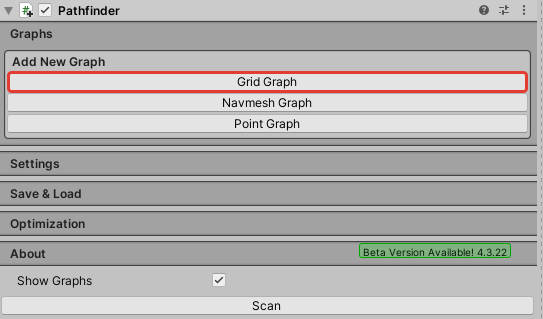# How to add a new Grid Graph

Hello! In my project, I generate a level randomly from prefabs of mini-locations. Each mini-location should have its own Grid Graph.I need to create a new Grid Graph with each mini-location. Thus, there should be many separate Grid Graphs at the level.How can I create a new grid graph through code?

Hi

Take a look at this tutorial: https://arongranberg.com/astar/docs/runtimegraphs.html

1 Like

This is what I was looking for. Thank! Please tell me how to change the type of Grid Graph from 3D to 2D?
I need now change those parameters…

I found a solution:

``````  void CreateGridGraph (Transform roomCenterTransform) {
//Holds all graph data
AstarData data = AstarPath.active.data;

//Creates a Grid Graph
GridGraph gridGraph = data.AddGraph (typeof (GridGraph)) as GridGraph;

//Switch to 2D mode
gridGraph.rotation = new Vector3 (-90, 270, 90);

//Setup a grid graph with some values
int width = 56;
int depth = 28;
float nodeSize = 0.5f;
gridGraph.SetDimensions (width, depth, nodeSize);

//Move Grid Graph
gridGraph.center = roomCenterTransform.position;

//Use 2D physics
gridGraph.collision.use2D = true;

//Diameter
gridGraph.collision.diameter = 1.5f;